# THE PLANE, INTER FIRST YEAR MATHEMATICS 1B PROBLEMS WITH SOLUTIONS

Mathematics 1B Intermediatesolutions for some problems are available here. These are very simple to understand.

Study the textbook lesson The Plane very well.

Observe the example problems and solutions given in the textbook

Textbook solutions for The Plane

Exercise 7(a)

You can also see

Inter Maths 1A textbook solutions

Inter Maths 1B textbook solutions

Inter Maths IIA textbook solutions

Inter Maths IIB textbook solutions

M

## THE PLANE, INTERMEDIATE 1ST YEAR MATHEMATICS 1B PROBLEMS WITH SOLUTIONS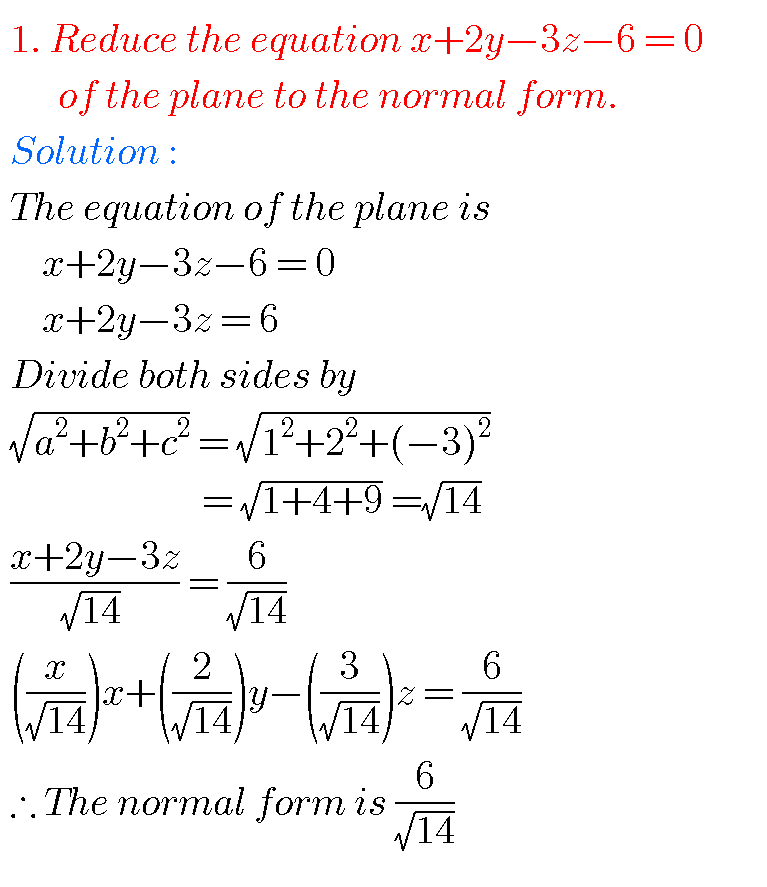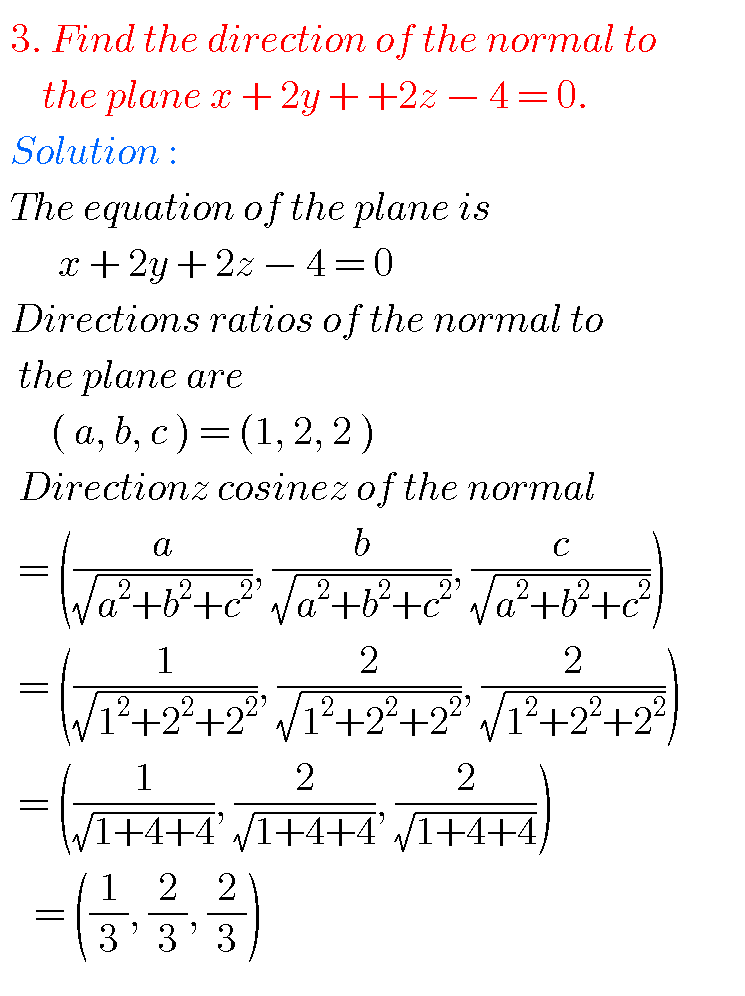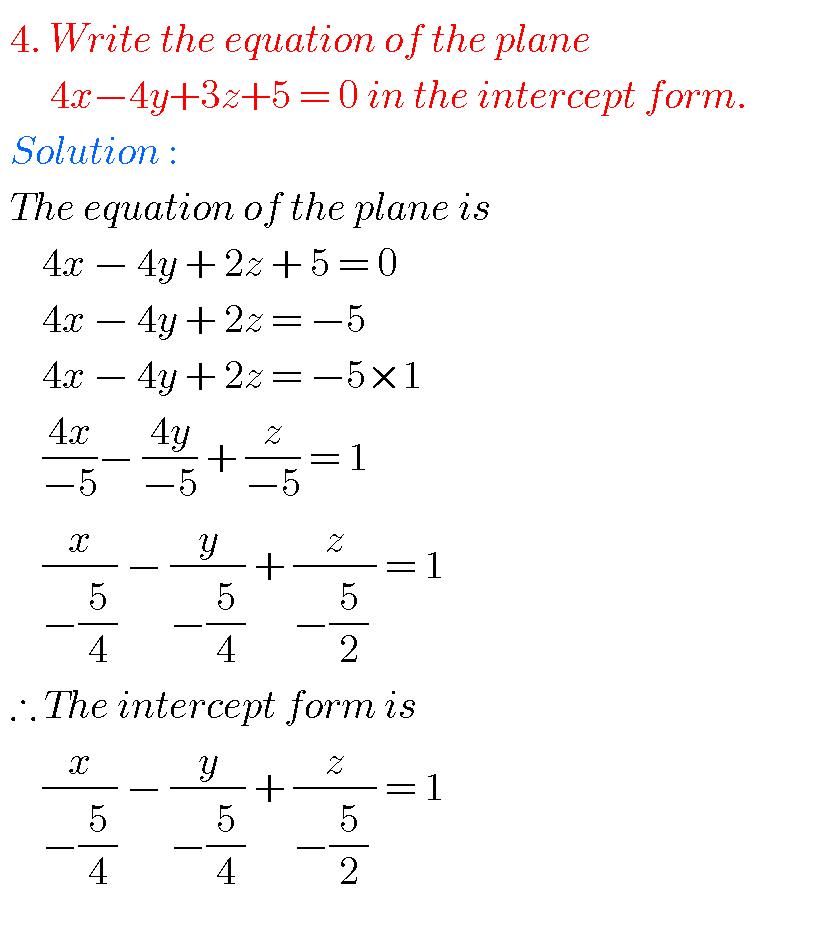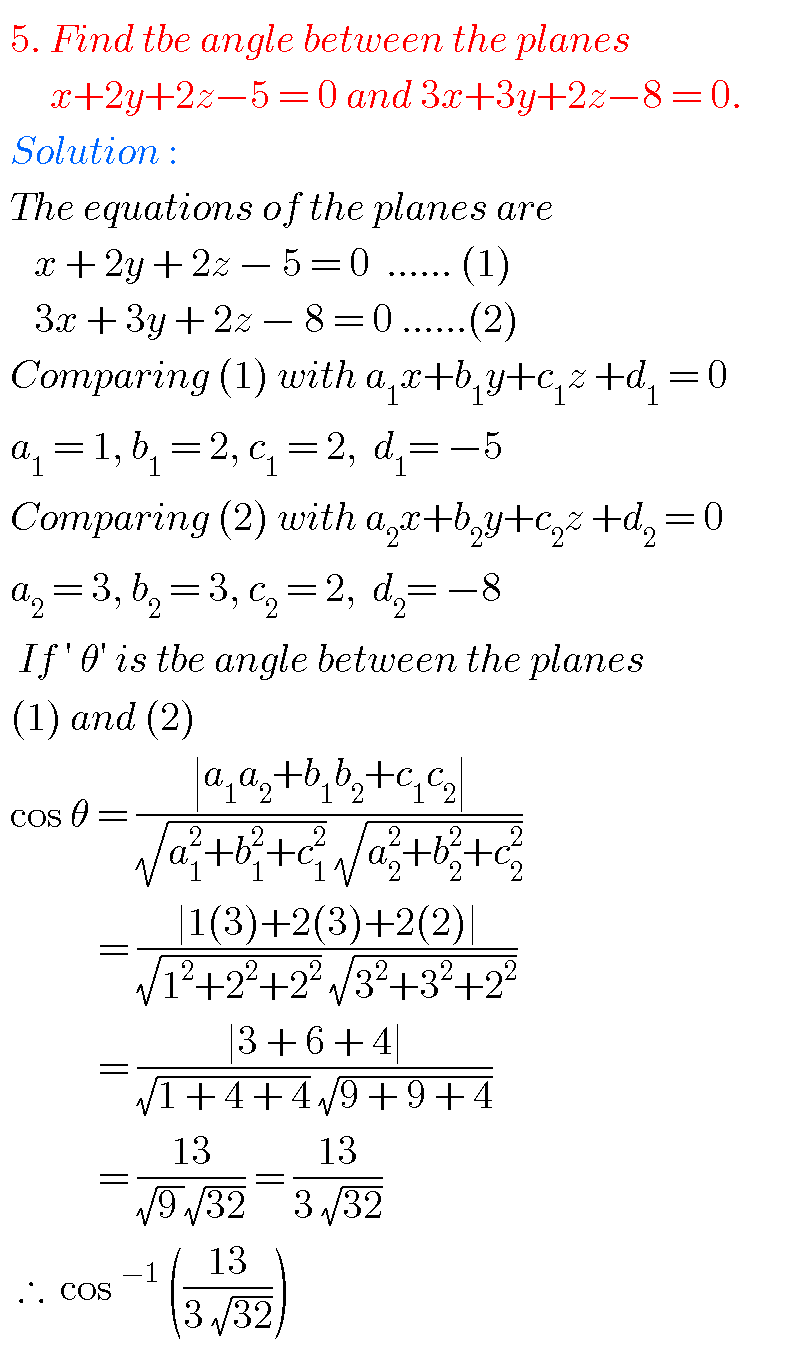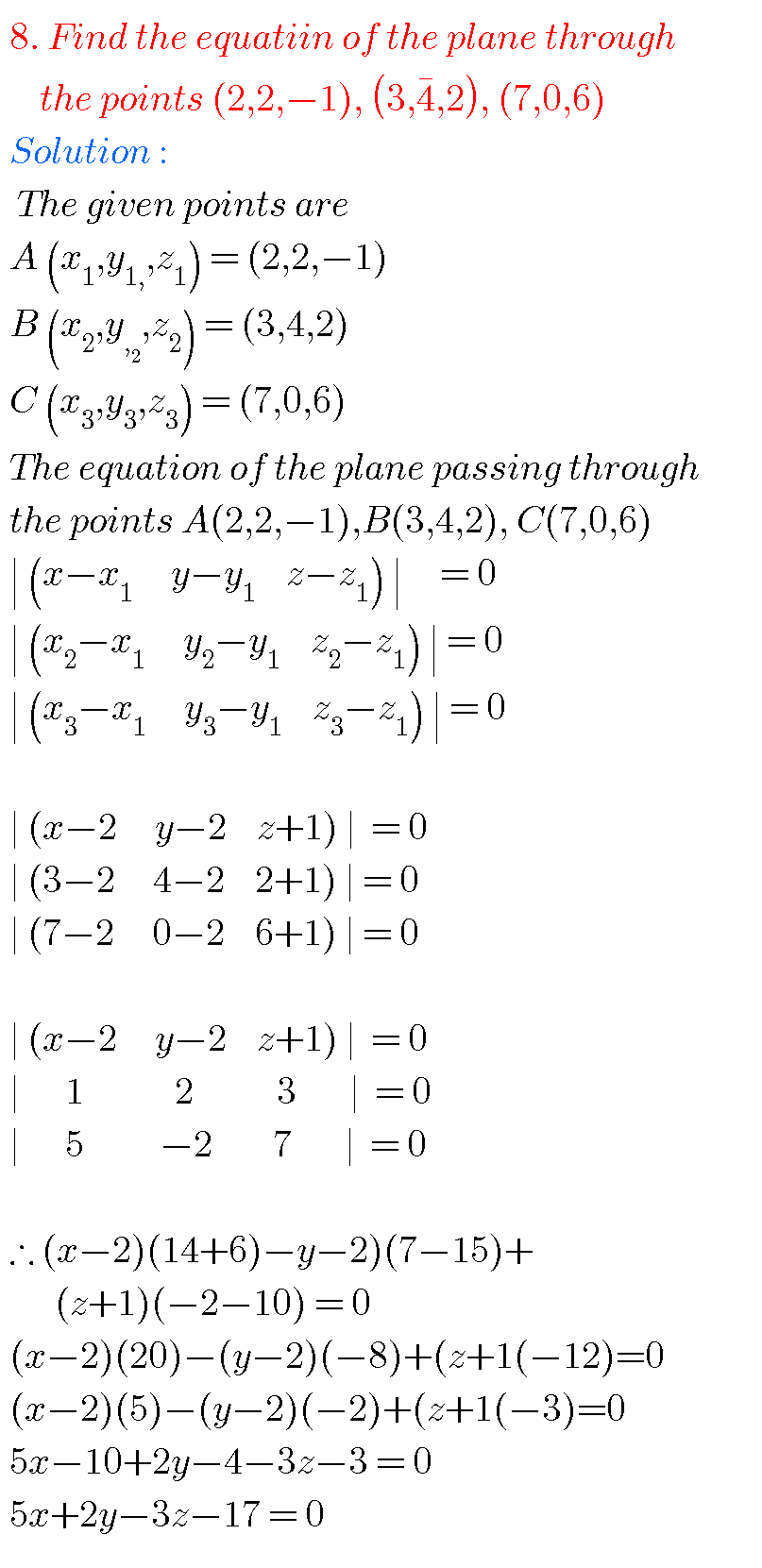Note : Observe the solutions and try them in your own methods.You can see the solutions for junior inter maths 1B

1Locus

Straight lines sa

Straight lines la

12. Rate measure

Inter maths trigonometry solutions

Maths real numbers solutions class 10

Ncert maths class 6 chapter 14 solutions

Nios maths 311 book 1 Sets 1.1 solutions